# Pre-Algebra - Polynomials: Division (Part 2)

This Math quiz is called 'Pre-Algebra - Polynomials: Division (Part 2)' and it has been written by teachers to help you if you are studying the subject at middle school. Playing educational quizzes is a fabulous way to learn if you are in the 6th, 7th or 8th grade - aged 11 to 14.

It costs only \$12.50 per month to play this quiz and over 3,500 others that help you with your school work. You can subscribe on the page at Join Us

A polynomial is two or more monomial numbers that are linked together in an equation by an addition sign (+), subtraction sign (-), a multiplication sign (x) or now a division sign (÷). In this quiz, we will be dealing with dividing a polynomial by a polynomial. It is a little bit harder than dividing a polynomial by a monomial but if you take it step by step, it will soon become easy. The easiest way to work out a polynomial divided by a polynomial number to find its solution is to use long division.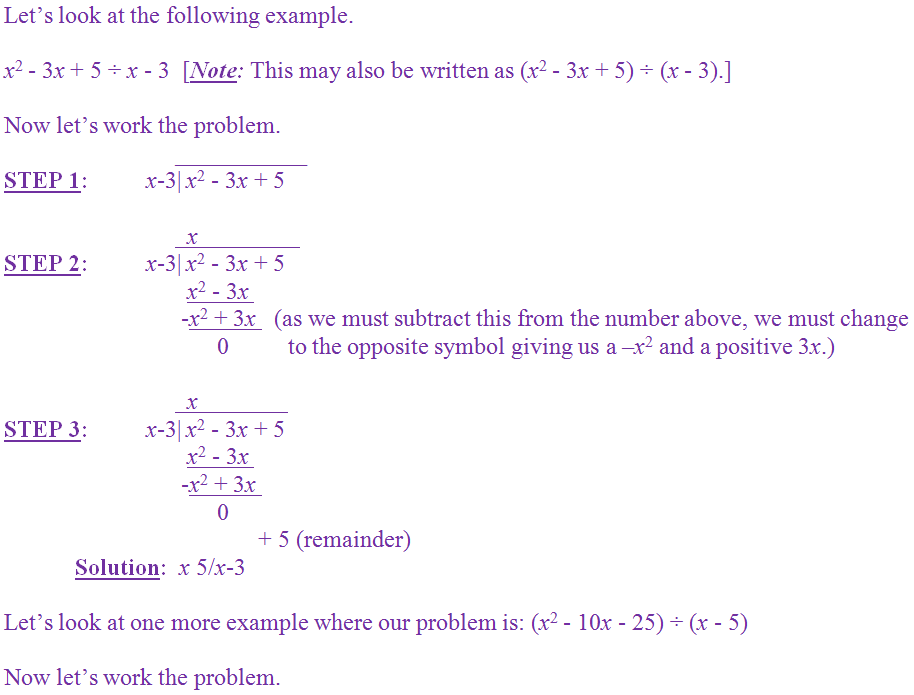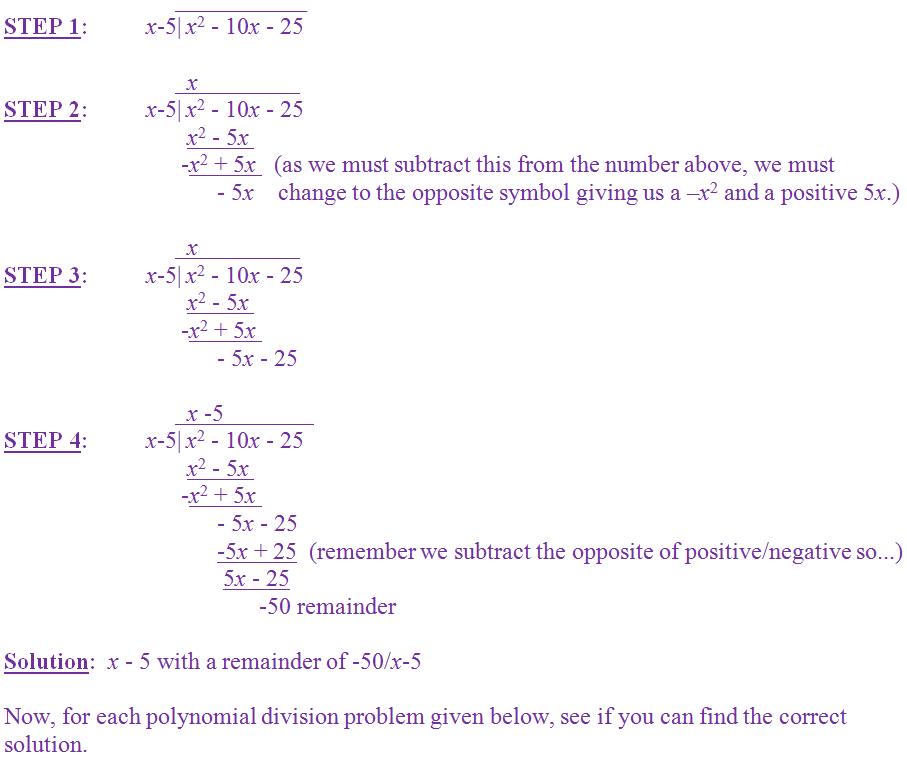Question 1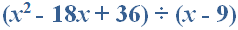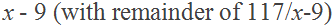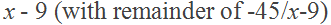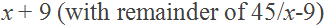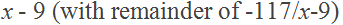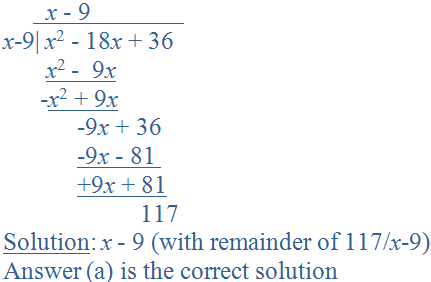Question 2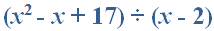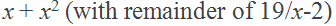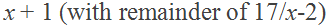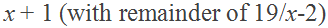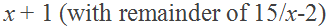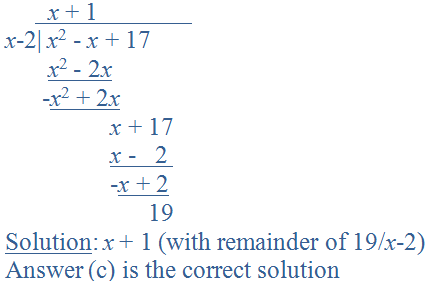Question 3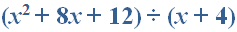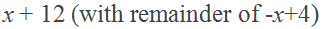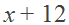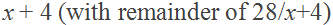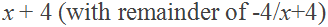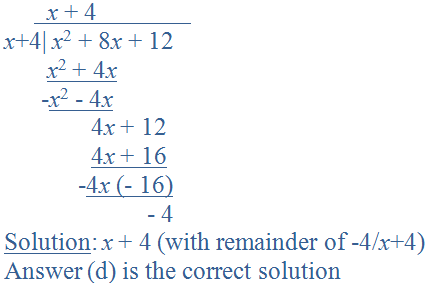Question 4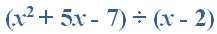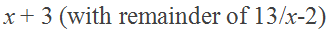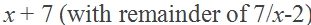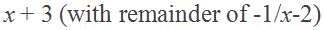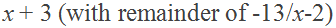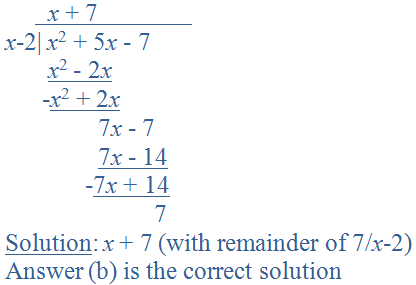Question 5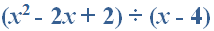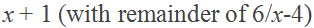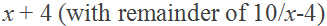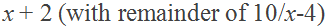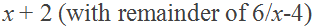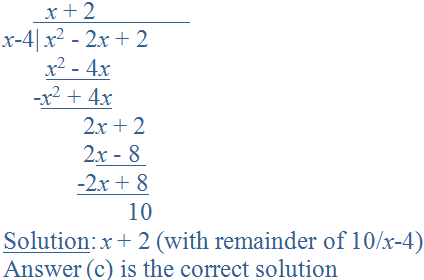Question 6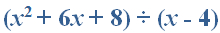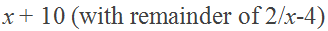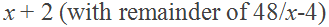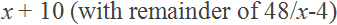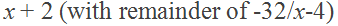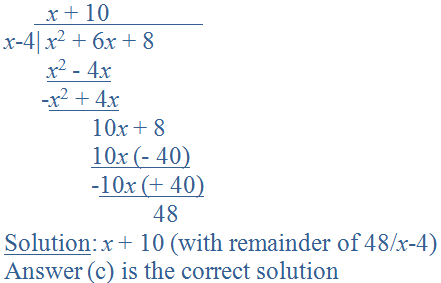Question 7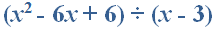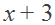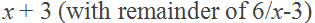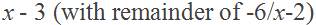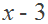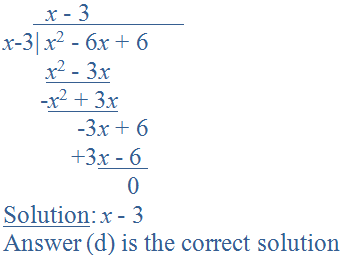Question 8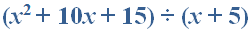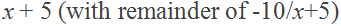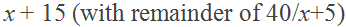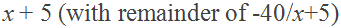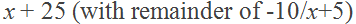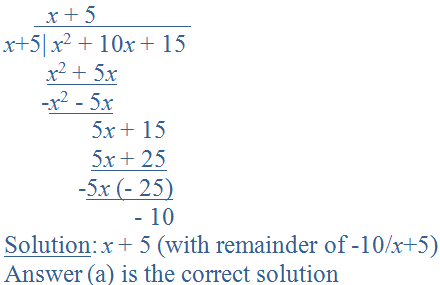Question 9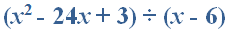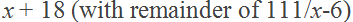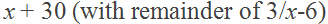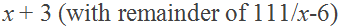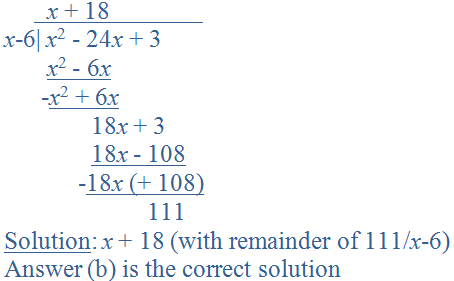Question 10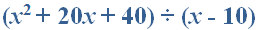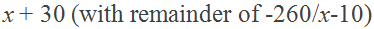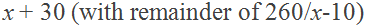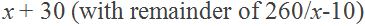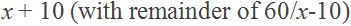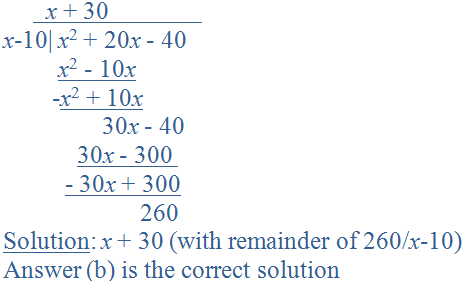Author:  Christine G. Broome